# Area Between Two Curves - Calculus

As we all know, integration means calculating the area by dividing the region into many elementary strips and then adding up these elemental areas. We can calculate the area bounded by a curve and a line between a given set of points at this stage. In the upcoming discussion, you will learn how to find the enclosed area between two curves in calculus with two cases and examples.

## What is the Area Between Two Curves?

We know that the area is the quantity used to express the region occupied by the two-dimensional shapes in the planar lamina. In calculus, the evaluate the area between two curves, it is necessary to determine the difference of definite integrals of a function. The area between the two curves or functions is defined as the definite integral of one function, say f(x), minus the definite integral of other functions, say g(x) with the lower and upper bounds as a and b, respectively. Thus, it can be represented as the following:

Area between two curves = ∫a[f(x) – g(x)] dx

## How to Find the Area Between Two Curves?

Case 1: Consider two curves y = f(x) and y = g(x), where f(x) g(x) in [a, b]. In the given case, the point of intersection of these two curves can be given as x = a and x = b, by obtaining the given values of y from the equation of the two curves.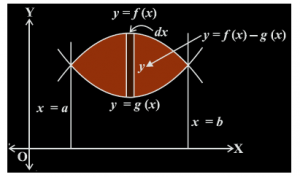Our aim is to find the enclosed area between the two given curves. In order to do so, a thin vertical strip of width dx is taken between the lines x = a and x = b as shown in the figure. The height of this vertical strip is given as f(x) – g(x). So, the elementary area of this strip dA can be given as [f(x) – g(x)]dx.

Now, we know that the total area is made up of vary large number of such strips, starting from x = a to x = b. Hence, the total enclosed area A, between the curves is given by adding the area of all such strips between a and b:

$$\begin{array}{l} A = \int\limits_{a}^b [f(x) – g(x)] dx\end{array}$$

The enclosed area between two curves can also be calculated in the following manner,

A = (area bounded by the curve y = f(x), x-axis and the lines x = a and x = b) – (area bounded by the curve y = g(x), x-axis and the lines x = a and x = b)

$$\begin{array}{l} A = \int\limits_{a}^b f(x)dx- \int\limits_{a}^b g(x)dx = \int\limits_{a}^b [f(x) – g(x)] dx \end{array}$$

where f(x)  g(x), in [a,b]

Case 2: Consider another case, when two curves y = f(x) and y = g(x) are given, such that f(x) g(x) between x = a and x = c and f(x) g(x) between x = c and x = b, as shown in the figure.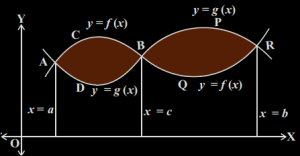In this case to calculate the total area between the two curves, the sum of the areas of the region ACBDA and BPRQB is calculated i.e.

$$\begin{array}{l} Total ~area = \int\limits_{a}^c [f(x) – g(x)] dx + \int\limits_{c}^b [g(x) – f(x)] dx\end{array}$$

### Area Between Two Curves Formula

Formula for area between two curves, integrating on the x-axis is given as:

$$\begin{array}{l}\mathbf{A=\int_{x_1}^{x_2}[f(x)-g(x)]dx}\end{array}$$

The function with the greater value of y for a given x is taken to be the upper function, i.e. f(x) and the function with the smaller value of y for a given x is taken to be the upper function, i.e. g(x). Also, it is possible that the upper and the lower functions can be different based on the different regions on the graph. In such cases, we need to calculate the area for the individual region.
Formula for area between two curves, integrating on the y-axis is given as:

$$\begin{array}{l}\mathbf{A=\int_{y_1}^{y_2}[u(y)-v(y)]dy}\end{array}$$

The function with the greater value of x for a given y is taken to be the right function, i.e. u(y) and the function with the smaller value of x for a given y is taken to be the left function, i.e. v(y). Also, it is possible that the left and right functions can be different based on the different regions on the graph. In such cases, we need to calculate the area for the individual region.

### Area Between Two Curves Examples

Let us consider an example that will give a better understanding.

Example: 1

Find the area of the region bounded by the parabolas y = x2 and x = y2.

Solution:

When the graph of both the parabolas is sketched we see that the points of intersection of the curves are (0, 0) and (1, 1) as shown in the figure below.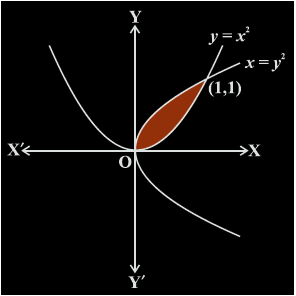So, we need to find the area enclosed between these points which would give us the area between two curves. Also, in the given region as we can see,

y = x= g(x)

and

x = y2

or, y = √x = f(x).

As we can see in the given region,

The area enclosed will be given as,

$$\begin{array}{l} A = \int\limits_{0}^1 [f(x) – g(x)] dx \end{array}$$

$$\begin{array}{l} = \int\limits_{0}^1 [\sqrt{x} – {x}^{2}] dx \end{array}$$

$$\begin{array}{l} = [\frac{2}{3}{x}^{3/2} -\frac{{x}^{3}}{3}{]}_{0}^{1}\end{array}$$

$$\begin{array}{l} = \frac{2}{3}- \frac{1}{3}\end{array}$$

$$\begin{array}{l} = \frac{1}{3} sq.units\end{array}$$

Example 2:

Find the area bounded by curves (x – 1)2 + y2 = 1 and x2 + y2 = 1.

Solution:

Given equations of curves:

x2 + y2 = 1 ….(i)

(x – 1)2 + y2 = 1 ….(ii)

From (i),

y2 = 1 – x2

By substituting it in equation (2), we get;

(x – 1)2 + 1 – x2 = 1

On further simplification

(x – 1)2 – x2 = 0

Using the identity a2 – b2 = (a – b)(a + b),

(x – 1 – x) (x – 1 + x) = 0

-1(2x – 1) = 0

– 2x + 1 = 0

2x = 1

x = 1/2

Using this in equation (1) we get;

y = ± √3/2

Thus, both the equations intersect at point A (1/2, √3/2) and B (1/2, -√3/2).

Also, (0, 0) is the centre of first circle and radius 1

Similarly, (1, 0) is the centre of second circle and radius is 1.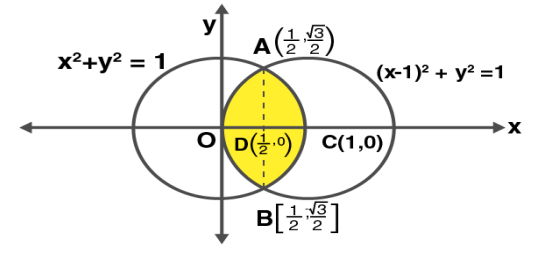Here, both the circles are symmetrical about x-axis and the required area is shaded here.

So, the required area = area OACB

= 2 (area OAC)

= 2 [area of OAD + area DCA]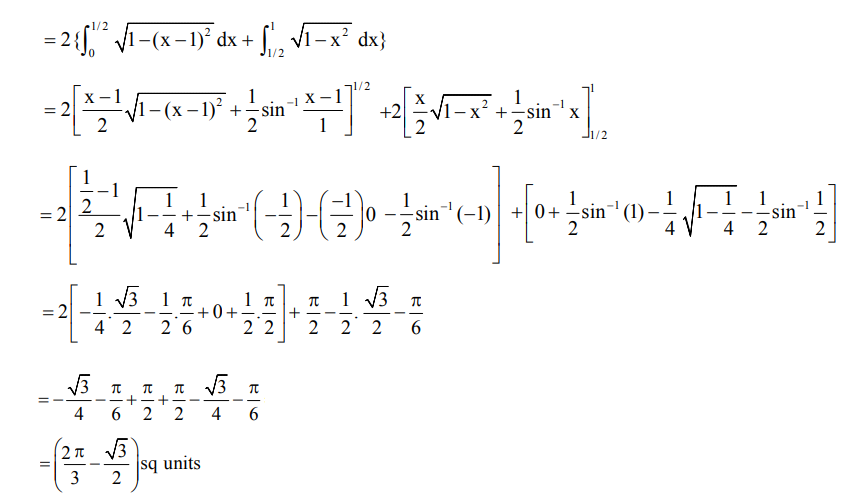### Area Between Two Curves Problems

Go through the practice problems given below to understand more about the method of finding the area of between two curves.

1. Find the area bounded by two curves x2 = 6y and x2 + y2 = 16.
2. Find the area of the region enclosed between the two circles: x2 + y2 = 4 and (x – 2)2 + y2 = 4.
3. Draw a rough sketch of the region {(x, y): y2 ≤ 3x, 3x2 + 3y2 ≤ 16} and find the area enclosed by the region, using the method of integration.